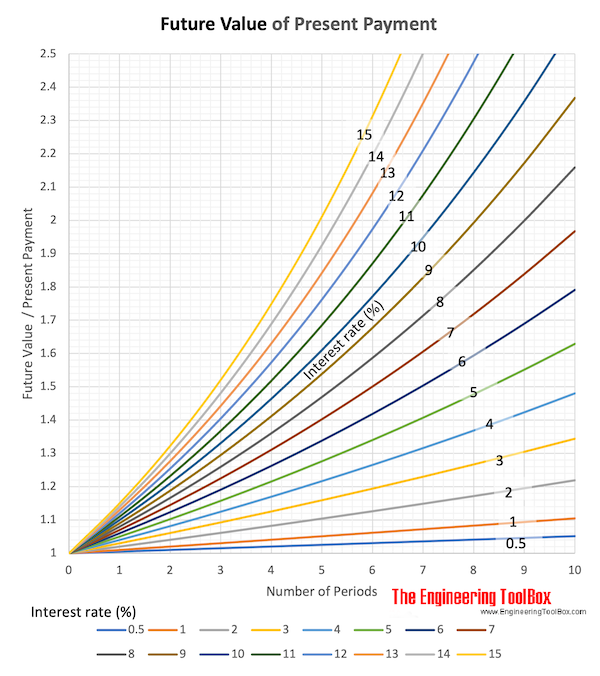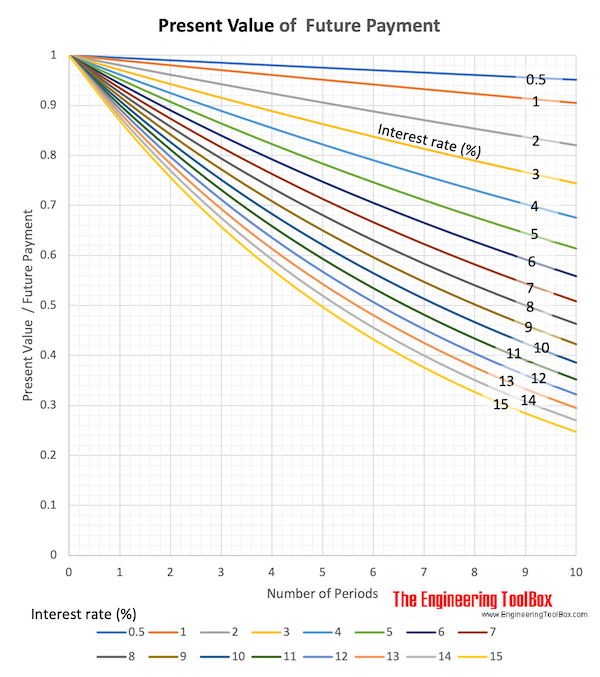Engineering ToolBox - Resources, Tools and Basic Information for Engineering and Design of Technical Applications!

# Interest Formulas

## Future value of single cash flow.

### Single Cash Accumulation - Future Value

The accumulated value of a present sum invested at a given interest rate after some time can be expressed as

F = P (1 + i)n         (1)

where

F = accumulated value in the future- Future Value

P = principal or present sum invested

i = interest rate per period

n = number of interest periods

The factor "(1 + i)n" is known as the "single payment compound amount factor".

#### Example - Accumulated amount

An amount of 1000 is invested at interest rate 10% (0.1) per year for 10 years. The accumulated amount can be calculated like

F = 1000 (1 + 0.1)10

= 2594#### Future Value Calculator

Present Value

no. of periods

interest rate per period

### Discounting Process - Present Value

The Present Value - or Present Worth - of a future cash flow can be calculated with the discounting process and is expressed like

P = F / (1 + i)n         (2)

where

P = present value

F = future cash flow

i = discount rate or discounting factor

n = numbers of periods

The factor "1 / (1 + i)n" is known as the "single payment present worth factor".

#### Example - The present value of a future sum

A sum of 1000 is paid after 10 years. The discount rate is 10% (0.1) per year. The present value of the future payment can be calculated like

P = 1000 / (1 + 0.1)10

= 386#### Present Value Calculator

Present Value

no. of periods

interest rate per period

## Related Topics

• Economics - Engineering economics - cash flow diagrams, present value, discount rates, internal rates of return - IRR, income taxes, inflation.

## Engineering ToolBox - SketchUp Extension - Online 3D modeling!

Add standard and customized parametric components - like flange beams, lumbers, piping, stairs and more - to your Sketchup model with the Engineering ToolBox - SketchUp Extension - enabled for use with the amazing, fun and free SketchUp Make and SketchUp Pro .Add the Engineering ToolBox extension to your SketchUp from the SketchUp Pro Sketchup Extension Warehouse!

Translate

## Privacy

We don't collect information from our users. Only emails and answers are saved in our archive. Cookies are only used in the browser to improve user experience.

Some of our calculators and applications let you save application data to your local computer. These applications will - due to browser restrictions - send data between your browser and our server. We don't save this data.

## Citation

• Engineering ToolBox, (2008). Interest Formulas. [online] Available at: https://www.engineeringtoolbox.com/interest-formulas-d_1230.html [Accessed Day Mo. Year].

Modify access date.

. .

#### Scientific Online Calculator6 24